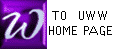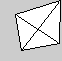a Simplex
SIMPLEX METHOD OUTLINE FOR
STANDARD MAXIMIZING PROBLEMS

INDEX
OF
TERMS
 PIVOT Constraint Objective Function Simplex Tableau Standard Maximizing Problem Hopping phase I Objective row Ratios (Quotients) Identity Sub-Matrix (ISM) Indicator z-column basic solution Final Tableau pivoting , pivot operation

 The simplex method changes constraints (inequalities) to equations in linear programming problems, and then solves the problem by matrix manipulation. The solution set for the altered problem is of higher dimension than the solution set of the original problem, but it is easier to study with matrices.

Reference : What is a STANDARD MAXIMIZING PROBLEM?

Reference : An example of SIMPLEX METHOD for a standard problem is available.

 STEP 1
 Rewrite each constraint (inequality) as an equation (Rolf 8th ed., pg. 276) : See example?

 STEP 2
Write the revised problem as a tableau, with the objective row (= bottom row) consisting of negatives of the coefficients of the objective function z ; z will be maximized. The lower right corner is the value of z when x, y,... are zero ; thus, z usually starts out as zero. See example?
 Reference: Some books (such as Rolf 8th ed., pg. 279) use an extra "z-COLUMN" which never changes and will be omitted in Prof McFarland's class. If you wish to use a z-COLUMN, this is OK: nothing else will be affected either way.

Definition
 The IDENTITY SUB-MATRIX (ISM) is an identity matrix located in the slack variable columns of the starting tableau, but moving to other columns during simplex method. This definition is not in Rolf.
Definition
 A BASIC SOLUTION for a tableau is a corner point of the (unseen) solution set of the original system of inequalities. For each tableau, the coordinates of its basic solution are as follows (Rolf 8th ed., pg. 287, or See example?): [a] All variables not associated with the ISM are set equal to zero. [b] Evaluate other variables by reading each tableau row as an equation.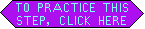Simplex method will move the ISM, one column at a time; after each such move, we arrive at (or "hop" to) a new corner point (basic solution) with bigger objective value. Since the solution set has only finitely many corners, this process ultimately yields the biggest value of the objective function.

 An INDICATOR (for standard maximizing problems) is a number in the bottom (objective) row of a tableau, excluding the rightmost number. Thus here, the INDICATOR ROW is the bottom row, but for non-standard problems the indicator row will be a different row.

FIRST FIND THE PIVOT: (Rolf 8th ed., pg. 294)

 STEP 3
The pivot column is that column containing the most negative indicator. If no indicator is negative, the tableau is a FINAL TABLEAU : see step 8.

 STEP 4
Form RATIOS (quotients) for each row: divide the right-most number by the number in the pivot column of that row.

 STEP 5
 The PIVOT ROW is the row with the smallest NON-NEGATIVE ratio (quotient). Note that 0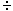(+1) and 0(-1) are both numerically zero, but in calculating RATIOS, consider 0(+1) as positive (OK), and 0(-1) as negative (not OK).

"HOPPING" : moving the identity sub-matrix (ISM), hence moving to a new corner point.

 STEP 6
Apply a pivot operation (Rolf 8th ed., pg. 98) to the tableau, including the bottom (objective) row. The pivot column will become a column of a new ISM in the new tableau. Note which column is replaced, and where the new ISM is located ; its columns may not be in the usual order: not to worry. Check out the PIVOT ENGINE to speed up practice.

 STEP 7
 (optional) Note the new basic solution (corner point) for the new tableau.

 STEP 8
If all indicators (in the bottom row) are non-negative,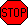: your tableau is a FINAL TABLEAU. The basic solution of step 7 is the maximal solution you have been seeking! Note that if you correctly reached this step from a Non-Standard Problem, then all right-side numbers above the objective row will also be non-negative.

 STEP 9
Otherwise, if some indicator remains negative, repeat steps 3 through 9 WITH YOUR NEW TABLEAU. After a finite number of such repetitions (usually 2-3), simplex method must terminate at step 8.